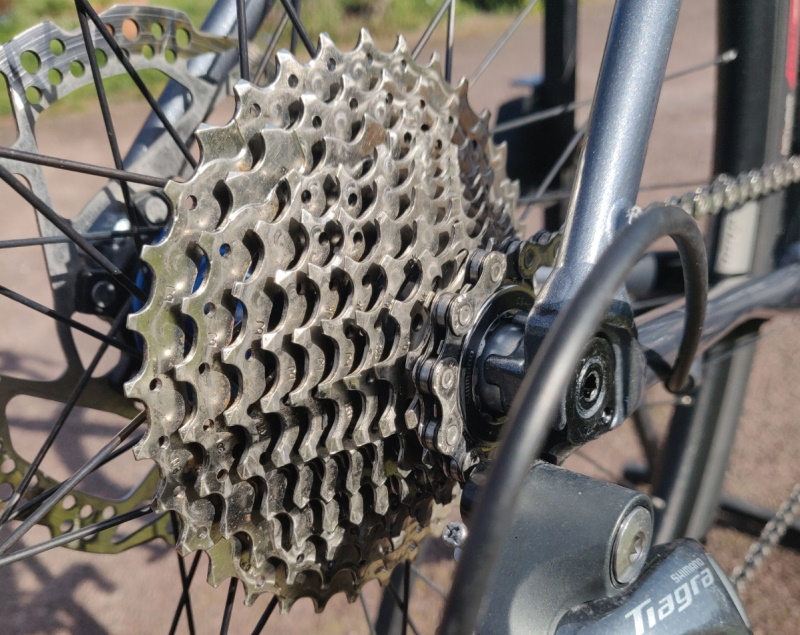# Making Sense of Gear RatiosYou’ve probably heard people talking about changing their gear ratios for a higher or lower gear to help with riding on the flat or climbing hills.

You will have also probably hear people saying things like they swapped from 52 x 13 to 50 x 14 but what does it all mean?

As far as bicycle gear ratios are concerned

• A low gear is an easier one to pedal and makes getting up hills easier.
• A high gear is harder to pedal but generates more distance and thus speed per pedal revolution.

To work out which combination of chainring / cassette is higher or lower it’s simple maths.  Just divide the number of teeth in the chainring by the number of teeth in the required cassette sprocket. E.g. in our two examples at the top of the page 52÷13 = 4 and 50÷14= 3.5714. This means that the for each turn of the pedals the wheel turns 4 times and 3.5714 times respectively. All things being equal, such as terrain, wheel size etc the smaller the number the lower the gear and the easier to pedal.

This then becomes difficult to compare when bicycle setups are different and this is where ‘Gear inches’ can help. Gear Inches is a throwback to the penny farthing when the pedals were fixed to the wheel of the bicycle. Each pedal revolution was directly relatable to the size of the wheel – one pedal revolution = one wheel revolution. As with gear ratios, the smaller the number the easier it is to pedal, but less distance travelled per revolution.

The calculation for gear inches is simply just an extension of the gear ratio calculation. Using our 52 x 13 gear ratio above  and a fairly typical 700x28c road wheel configuration which has a diameter of around 26.7 inches the calculation is to take the gear ratio and multiply by the wheel diameter so (52÷13) x 26.7 = 106.8 inches.

This method can be useful if you are trying to evaluate different setups where the wheel sizes may vary.

The final extension of this theory is to work out how far you will travel for each pedal revolution. So using our 52/13 and 700x28c example above multiply the 106.8 inches by pi (3.14159) to give 335.52 inches or 27.96 feet per pedal revolution.